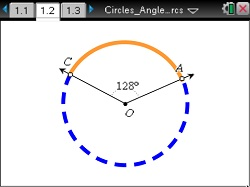### Mathematics lessons for IB® Diploma Programme

Applications and Interpretation | Geometry and Trigonometry## Circles: Angles and Arcs

### Activity Overview

This lesson involves manipulating endpoints of an arc, manipulating an inscribed angle, and manipulating the vertex of an angle intercepting an arc. As a result students will:

• Use visualization to understand the definitions of central angle, intercepted arc, and minor and major arcs.
• Infer that the sum of the measures of minor and major arcs is 360°, that two inscribed angles intercepting the same arc have the same measure, and that the inscribed angle has half the measure of the central angle that intercepts the same arc.
• Deduce that the opposite angles of a quadrilateral inscribed in a circle are supplementary.

### Objectives

• Students will know the definitions of and identify central angles, major and minor arcs, intercepted arcs, and inscribed angles of a circle.
• Students will determine and apply the following relationships: · Two inscribed angles intercepting the same arc have the same measure. · An inscribed angle measure of 90° results in the endpoints of the intercepted arc lying on a diameter. · The measure of an angle inscribed in a circle is half the measure of the central angle that intercepts the same arc.

### Vocabulary

• Central angle
• Inscribed angle
• Major arc
• Minor arc
• Intercepted arc